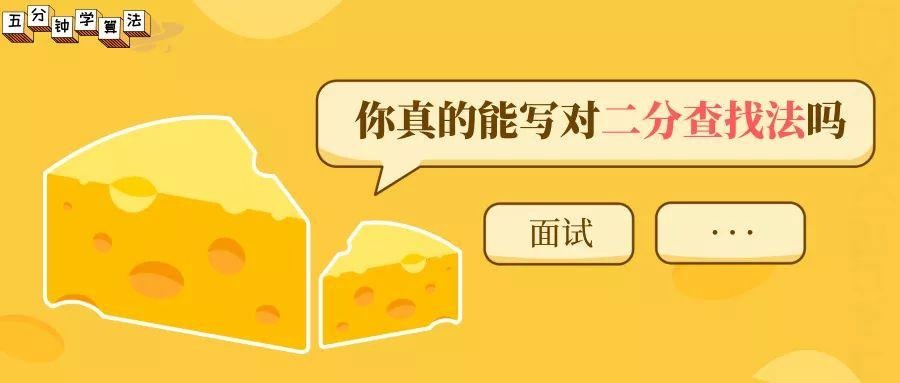### 前言科普

2019 年的你，在面试的过程中能手写出没有 bug 的二分查找法么？

### 二分查找法代码

public static int binary(int[] arr, int data) {        int min = 0;        int max = arr.length - 1;        int mid;        while (min <= max) {            mid = (min + max) / 2;            if (arr[mid] > data) {                max = mid - 1;            } else if (arr[mid] < data) {                min = mid + 1;            } else {                return mid;            }        }        return -1;    }

mid = (min + max) / 2;

public static int binary(int[] arr, int data) {        int min = 0;        int max = arr.length - 1;        int mid;        while (min <= max) {            // 防止溢出            mid =  min + (max - min) / 2;            if (arr[mid] > data) {                max = mid - 1;            } else if (arr[mid] < data) {                min = mid + 1;            } else {                return mid;            }        }        return -1;    }

 public static int binary(int[] arr, int data) {        int min = 0;        int max = arr.length - 1;        int mid;        while (min <= max) {            // 无符号位运算符的优先级较低，先括起来            mid =  min + ((max - min) >>> 1);            if (arr[mid] > data) {                max = mid - 1;            } else if (arr[mid] < data) {                min = mid + 1;            } else {                return mid;            }        }        return -1;    }